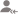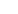trueAsked on 12 Jan Learn Practical Geometry

Draw an equilateral triangle one of whose sides is of length 7 cm.

Asked on 12 Jan Learn Practical Geometry

Draw a triangle ABC with AB = 6 cm, BC = 7 cm and CA = 8 cm. Using ruler and compass alone, draw (i)... read more
Draw a triangle ABC with AB = 6 cm, BC = 7 cm and CA = 8 cm. Using ruler and compass alone, draw (i) the bisector AD of ∠A and (ii) perpendicular AL from A on BC. Measure LAD. read less

Asked on 12 Jan Learn Practical Geometry

Draw △ABC in which AB = 3 cm, BC = 5 cm and ∠B = 70o.

Overview

Questions 20

Total Shares1 Followers

Asked on 12 Jan Learn Practical Geometry

Draw △ABC in which ∠A=70o. AB = 4 cm and AC= 6 cm. Measure BC.

Asked on 12 Jan Learn Practical Geometry

Find in square meters, the area of a square of side 16.5 dam.

Asked on 12 Jan Learn Practical Geometry

A wire is in the shape of a rectangle. Its length is 40 cm and breadth is 22 cm. If the same wire is... read more
A wire is in the shape of a rectangle. Its length is 40 cm and breadth is 22 cm. If the same wire is bent in the shape of a square, what will be the measure of each side. Also, find which side encloses more area? read less

Asked on 12 Jan Learn Practical Geometry

How many square meters of glass will be required for a window, which has 12 panes, each pane measuring 25 cm by 16 cm?

Asked on 12 Jan Learn Practical Geometry

A marble tile measures 10 cm x 12 cm. How many tiles will be required to cover a wall of size 3 m x 4... read more
A marble tile measures 10 cm x 12 cm. How many tiles will be required to cover a wall of size 3 m x 4 m? Also, find the total cost of the tiles at the rate of Rs 2 per tile. read less

Asked on 12 Jan Learn Practical Geometry

A table top is 9 dm 5 cm long 6 dm 5 cm broad. What will be the cost to polish it at the rate of 20 paise... read more
A table top is 9 dm 5 cm long 6 dm 5 cm broad. What will be the cost to polish it at the rate of 20 paise per square centimetre? read less

Asked on 12 Jan Learn Practical Geometry

A room is 9.68 m long and 6.2 m wide. Its floor is to be covered with rectangular tiles of size 22 cm... read more
A room is 9.68 m long and 6.2 m wide. Its floor is to be covered with rectangular tiles of size 22 cm by 10 cm. Find the total cost of the tiles at the rate of Rs 2.50 per tile. read less

UrbanPro.com helps you to connect with the best in India. Post Your Requirement today and get connected.

x

X

### Find Tutors, Trainers & Institutes near you

Post requirement and connect with the tutors in your locality

• Select the best Tutor
• Book & Attend a Free Demo
• Pay and start Learning### Want to learn something New?

Find best tutors, trainers & institutes near you on UrbanProUrbanPro.com is India's largest network of most trusted tutors and institutes. Over 55 lakh students rely on UrbanPro.com, to fulfill their learning requirements across 1,000+ categories. Using UrbanPro.com, parents, and students can compare multiple Tutors and Institutes and choose the one that best suits their requirements. More than 7.5 lakh verified Tutors and Institutes are helping millions of students every day and growing their tutoring business on UrbanPro.com. Whether you are looking for a tutor to learn mathematics, a German language trainer to brush up your German language skills or an institute to upgrade your IT skills, we have got the best selection of Tutors and Training Institutes for you. Read more